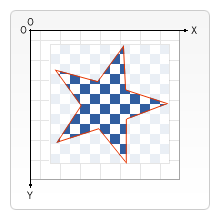# 组合 Compositing

## `globalCompositeOperation`

`globalCompositeOperation = type`

## 裁切路径裁切路径和普通的 canvas 图形差不多，不同的是它的作用是遮罩，用来隐藏不需要的部分。如右图所示。红边五角星就是裁切路径，所有在路径以外的部分都不会在 canvas 上绘制出来。

`clip()`

### `clip` 的例子

js

``````function draw() {
var ctx = document.getElementById("canvas").getContext("2d");
ctx.fillRect(0, 0, 150, 150);
ctx.translate(75, 75);

// Create a circular clipping path
ctx.beginPath();
ctx.arc(0, 0, 60, 0, Math.PI * 2, true);
ctx.clip();

// draw background

ctx.fillRect(-75, -75, 150, 150);

// draw stars
for (var j = 1; j < 50; j++) {
ctx.save();
ctx.fillStyle = "#fff";
ctx.translate(
75 - Math.floor(Math.random() * 150),
75 - Math.floor(Math.random() * 150),
);
drawStar(ctx, Math.floor(Math.random() * 4) + 2);
ctx.restore();
}
}
function drawStar(ctx, r) {
ctx.save();
ctx.beginPath();
ctx.moveTo(r, 0);
for (var i = 0; i < 9; i++) {
ctx.rotate(Math.PI / 5);
if (i % 2 == 0) {
ctx.lineTo((r / 0.525731) * 0.200811, 0);
} else {
ctx.lineTo(r, 0);
}
}
ctx.closePath();
ctx.fill();
ctx.restore();
}
``````